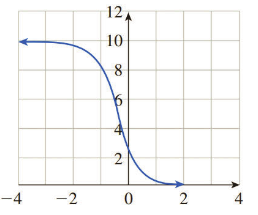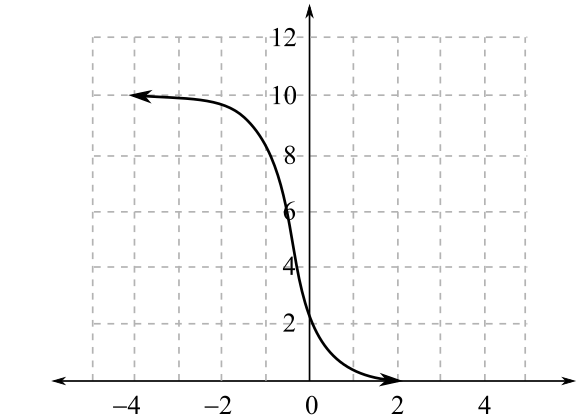Chapter 2.4, Problem 14EFinite Mathematics and Applied Cal...

7th Edition
Stefan Waner + 1 other
ISBN: 9781337274203

Solutions

Chapter
SectionFinite Mathematics and Applied Cal...

7th Edition
Stefan Waner + 1 other
ISBN: 9781337274203
Textbook Problem

In Exercises 11-16, choose the logistic function that best approximates the given curve.(A) f ( x ) = 10 1 + 3 ( 1.01 ) − x (B) f ( x ) = 8 1 + 7 ( 0.1 ) − x (C) f ( x ) = 10 1 + 3 ( 0.1 ) − x

To determine

The correct logistic function f

from the given choices for the following curve,(A). f(x)=101+3(1.01x)

(B). f(x)=81+7(0.1x)

(C). f(x)=101+3(0.1x)

Explanation

Given Information:

The provided curve is,

The given choices for the correct logistic function are,

(A). f(x)=101+3(1.01x)

(B). f(x)=81+7(0.1x)

(C). f(x)=101+3(0.1x)

The form of the logistic function is:

f(x)=N1+A(bx)

Where constants N,A

and b

are non-zero (A,bare positive and b1).

There are certain properties of the curve represented by the logistic curve, y=N1+A(bx)

1.

The graph of the function formed is S-shaped which is formed by sandwiching between the horizontal lines y=0 and y=N, which means the limiting value of the function is N.

2.

If b>1, graph rises, if b<1, the graph falls.

Now, the provided curve is,

It is clear from the curve that the function f

has limiting value 10, which means the value of constant N=10.

Now, consider the general logistic equation,

f(x)=N1+A(bx)

The graph illustrates that the given curve passes through the point, (0,2.5)

Substitute 10

for N, 0

for x and 2.5

for f(x) in f(x)=N1+A(bx):

2.5=101+A(b(0))2.5=101+A

Multiply both sides by (1+A):

2.5(1+A)=101+A(1+A)2

Still sussing out bartleby?

Check out a sample textbook solution.

See a sample solution

The Solution to Your Study Problems

Bartleby provides explanations to thousands of textbook problems written by our experts, many with advanced degrees!

Get Started

1340 Calculate y. xy4+x2y=x+3y

Calculus (MindTap Course List)

What are the two requirements for a random sample?

Statistics for The Behavioral Sciences (MindTap Course List)

Simplify each radical expression. (32)2

Trigonometry (MindTap Course List)

The area of the region bounded by , , and r = sec θ is:

Study Guide for Stewart's Multivariable Calculus, 8th

The general solution to dydt=ky is: a) y(t) = y(0)ekt b) y(t) = y(k)et c) y(t) = y(t)ek d) y(t) = ey(0)kt

Study Guide for Stewart's Single Variable Calculus: Early Transcendentals, 8th